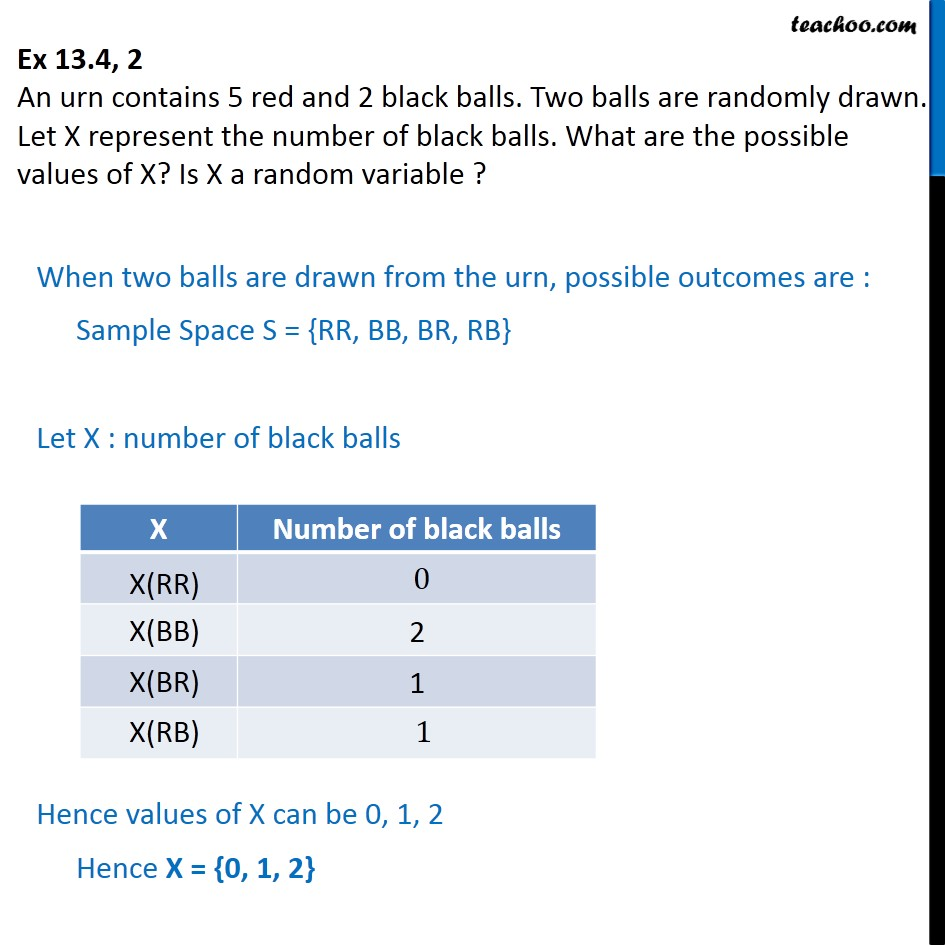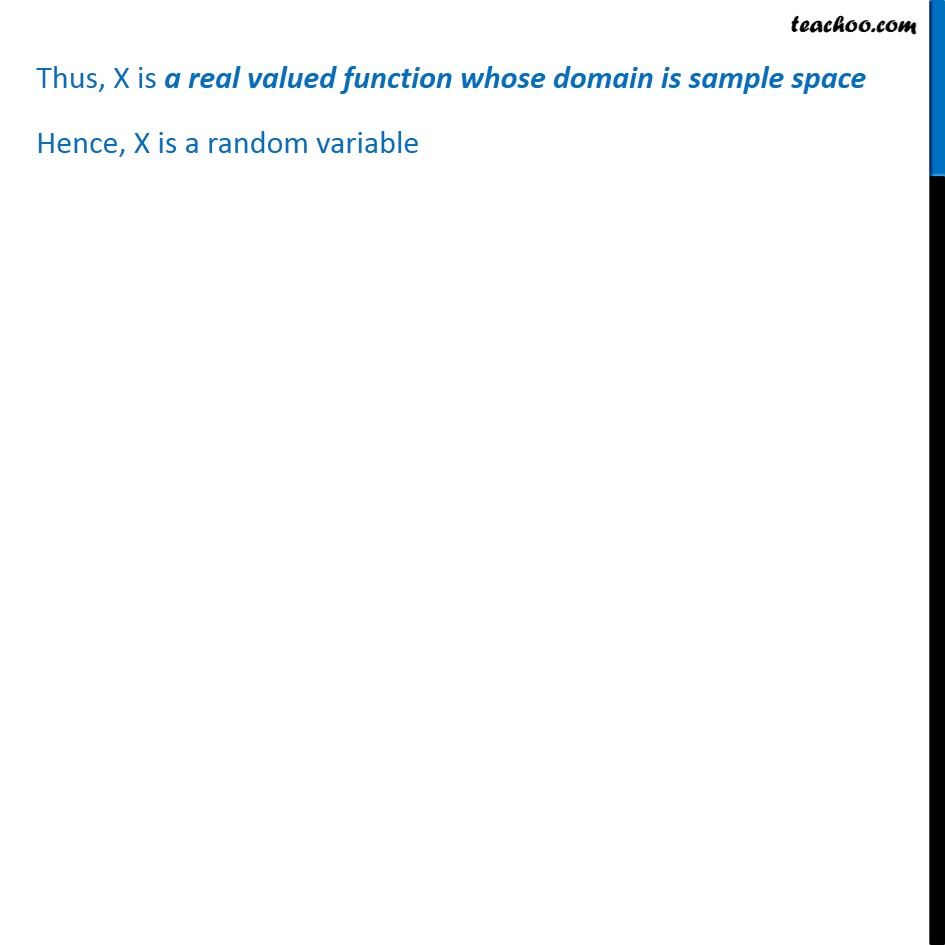Random Variable - Defination

Chapter 13 Class 12 Probability (Term 2)
Concept wise### Transcript

Ex 13.4, 2 An urn contains 5 red and 2 black balls. Two balls are randomly drawn. Let X represent the number of black balls. What are the possible values of X? Is X a random variable ? When two balls are drawn from the urn, possible outcomes are : Sample Space S = {RR, BB, BR, RB} Let X : number of black balls Hence values of X can be 0, 1, 2 Hence X = {0, 1, 2} Thus, X is a real valued function whose domain is sample space Hence, X is a random variable